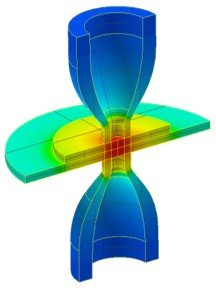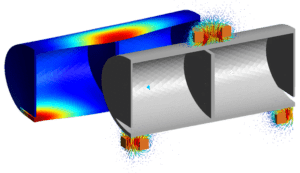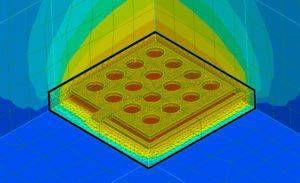# Available features

Here, you will find a broad list of physics and couplings available in the OOFELIE::Mutiphysics Solver.

Physics

The following physical fields are available in OOFELIE::Multipysics.

MechanicalThermalElectricalAcousticsFluidsTopology OptimizationMechanisms

• Static and transient
• Linear and non-linear
• Modal
• Real and complex
• Wet-mode shapes
• Harmonic
• Linear buckling
• Random vibrations
• Strength assessment
• Topology optimization
• Density based (SIMP)
• Iso- and orthotropic materials:
• Viscous, viscoelastic and structural damping
• Elasto-plastic, hyper-elastic non-linear materials
• Perfectly matched layers (PML)
• 3D modelling
• (Oriented) Volume, membrane, shell, rod, beam, lumped mass elements
• Large displacements & pre-stress
• Identical nodes, contact, perfect/non-perfect mechanical gluing, rigid body assemblies, mean
• Special effects:
• Coriolis
• Centrifugal
• 2-ways coupling with:
• Thermal field
• Electrical field
• 1-way coupling with optics

• Linear and non-linear
• Topology optimization
• Density based (SIMP)
• 3D modelling
• Identical nodes, perfect/non-perfect thermal gluing, thermal contact admittance
• 3D mutual radiation (ray tracing)
• Orbital model
• 2-ways coupling with
• Mechanical field
• Electrical field

• Static and transient;
• Linear and non-linear
• FEM / BEM coupling
• Dielectric materials
• Identical nodes
• Perfect/non-perfect electrical gluing
• RLC, electric dipole elements
• 2-ways coupling with
• Mechanical field
• Thermal field

• Modal and Harmonic
• FEM/BEM coupling
• Acoustic Surface
• Acoustic Rayleigh Surface
• Acoustic material
• Viscous, Structural Damping, Perfectly matched layers (PML)
• Absorbent panel with frequency dependent admittance and impedance
• Plane wave excitation, Point acoustic source, Prescribed normal displacement, velocity, acceleration
• 2-ways coupling with mechanical field

• Static and Transient
• Linear
• Non-linear
• Any gas or liquid material (Newtonian behaviour)
• 3D, 2D planar and axisymmetric modelling
• Symmetry planes
• Incompressible, Boussinesq Law, Compressible subsonic & supersonic (FINE™/FSI-OOFELIE), Inviscid, Laminar viscous, Turbulent viscous (FINE™/FSI-OOFELIE)
• Boundary conditions
• Velocity and temperature at inlet
• Pressure at outlet
• Boundary conditions
• Non-slip flow
• Surface heat flux or temperature at walls
• 2-ways coupling with mechanical and thermal fields

• Physics
• Mechanical
• Thermal
• Static analysis
• Gradient based method with MMA optimizer
• Density-based approach
• Adjoint variable method for sensitivities computation
• Multimaterial with or without void
• Manufacturing constraint:
• Minimum member size
• Overhang
• Filters on:
• Sensitivities
• Densities
• Various objective and constraint functions
• Volume (Total or On specific material)
• Total mass
• Mechanical compliance
• Thermal compliance
• Displacement on points
• Rotation on points
• Temperature on points
• Eigen frequencies
• Uncertainty quantification in the model related to
• Material properties specifications

• Non-linear transient analysis
• Rigid body
• Joints
• Hinge
• Cylindrical
• Prismatic
• Screw
• Universal
• Co-rotational approach
• Linear Super Element Models
• Multilayer multiphysics shell elements
• Mechanical mean
• Large rotations beam
• Controler definition
• PID
• LTI
• Preliminary support of FMU import
• Coupling with 3D mechanical elements

Couplings

The following couplings are accessible in OOFELIE::Multipysics.

Thermo-MechanicsOpto-Thermo-MechanicsCoupled Electrostatics-MechanicsPiezoelectricsPyro-PiezoelectricsVibroacouticsElectromagnetics – Low FrequencyElectro-Thermo-MechanicsElectromagnetics – High FrequencyHygro-Thermo-Mechanics

• Static and Transient
• Linear and non-linear
• Complex Modal and Harmonic
• 3D modelling
• Thermo-elastic iso- and orthotropic materials
• Thermo-elastic damping effect
• Thermal dependency of material properties
• 2-ways coupling with electric field
• 1-way coupling with optics

• Coupling with Zemax’ OpticStudio
Automated data exchange via in-memory dialog
• Description of surface deformations:
Zernike Standard or Fringe polynomials, grid of points, all aperture types
• Rigid body motion recognition and ability to export separately to Zemax’ OpticStudio
• Thermo-optic effect:
• Accounting for refractive index change as a function of Temperature.
• Computation and visualization of refractive index gradients inside lenses.
• Automated exportation to Zemax’ OpticStudio

• FEM/BEM coupling
• Mesh morphing
• Fast Multipole Methods for BEM
• Pre-stress
• Pull-in voltage computation

• Static and transient
• Linear and non-linear
• Modal and harmonic
• Piezoelectric materials:
• Hexagonal C6
• Trigonal D3
• Triclinic C1
• Perfectly matched layers (PML)
• (Oriented) Volume, membrane, shell
• 2-ways coupling with thermal
• 1-way coupling with optics

• Pyro- Piezoelectric materials:
• Hexagonal C6
• Trigonal D3
• Triclinic C1
• (Oriented) Volume
• 1-way coupling with optics

• Modal analysis
• Harmonic analysis coupled
• Complex direct
• Projection in coupled modal basis
• Projection in uncoupled modal bases
• Harmonic analysis uncoupled
• FMM: Fast Multipole Method
• Vibroacoustic Interaction using Incompatible meshes
• Node-based interpolation
• Projection interpolation
• 2-ways coupling with Pyropiezoelectric
• 1-way coupling with Electromagnetic

• Electro-technical hypotheses
• Static and Transient
• Linear and non-linear
• Harmonic
• 3D, 2D planar and axisymmetric modelling
• Magnetic non-conductor and conductor, isolator, Active and Passive conductor
• Permanent magnet
• Inductor
• Given current direction, Axi-symmetric
• Electromotive source
• Current driven
• Potential driven
• Infinite extension medium, electromagnetic wire
• Coupling with Mechanical (Laplace force) and Thermal (Joule effect) fields

• Static and Transient
• Linear and non-linear
• Thermoelectric conductor isotropic
• Thermo-elastic electric conductor isotropic
• Peltier, Seebeck , Thomson, Piezo-resistive effects
• Joule Heating
• Thermal dependency of Electrical and Thermal conductivity

• Electromagnetic wave prpagation based on FDTD
• Dissipative materials
• PLM
• Conforme mesh
• S-parameters

• Coupled three-field simulation

## Electrokinetic thermomechanics

• Conduction current computation
• Joule effect heating with thermomechanical coupling
• Steady state or transient analysis
• No magnetic field is considered## Electromagnetics - Low Frequency

• Magnetostatics (magnetic field constant in time)
• Source: Constant conduction current
• Source: Permanent magnet
• Electric field not influenced by magnetic field
• Magnetodynamics (magnetic field variable in time)
• Transient and harmonic analysis
• Electric and magnetic field are fully coupled
• Eddy current computation
• Couplings with mechanical field (Laplace force) and thermal field (Joule effect)## Electromagnetics - High Frequency

Full wave Maxwell’s Equations solver
• FDTD
• PML
• Tangent losses
• Near-field-to-far-field transformations

## Electrostatics mechanics

• Computation of electrostatic forces using FEM or BEM
• FEM/FEM coupling with mechanics.
• Mesh morphing is available to account of large displacements of the structure.
• FEM/BEM coupling with mechanics.
• Fast Multipole Method available to accelerate large scale applications.
• Understand the effect of pre-stesses on the response of the system.
• Computation of the Pull-in voltage.